### Home > INT2 > Chapter 10 > Lesson 10.1.1 > Problem10-13

10-13.

Calculate the area of each figure below. Show all work.

1.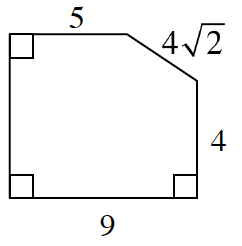Try breaking up the shape into two rectangles and one triangle as shown below.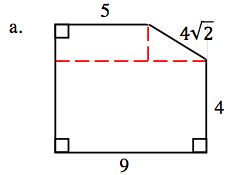If the bottom length is $9$ units, then what must be the total length of the longest dotted line? How does that help you determine the length of the bottom leg of the triangle?

With the given hypotenuse and side lengths, what could the angle measurements be for the triangle?
What do the angles say about the missing side length?

$64$ units

1.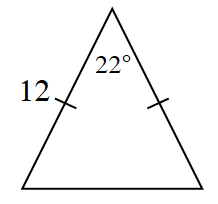Create two right triangles within the isosceles triangle.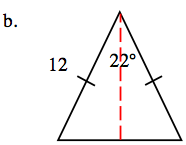Recall: $\sin \theta = \frac{\text{opp.}}{\text{hyp.}}$

1.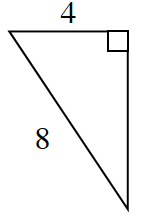See hints from parts (a) and (b).